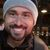# App Development

cancel
Showing results for
Did you mean:Partner

## Set Analysis limitation, bug or misunderstanding?

Hi there! 😃

I'm trying to calculate averages without outliers. I've used the FRACTILE function to determine which numbers are my outliers and exclude them from my AVG. Basically, the Function 1 (in this example) brings a value that is not the same when it is inside a set analysis condition, on the same tuple.

[Function 1] FRACTILE({<[QTD]-={0}>} [QTD],0.75)+(1.5*(FRACTILE({<[QTD]-={0}>} [QTD],0.75)-FRACTILE({<[QTD]-={0}>} [QTD],0.25)))
RESULT: 599,75

ROUND(AVG({<[QTD]-={">=\$(=FRACTILE({<[QTD]-={0}>} [QTD],0.75)+(1.5*(FRACTILE({<[QTD]-={0}>} [QTD],0.75)-FRACTILE({<[QTD]-={0}>} [QTD],0.25))))<=-214,25"}>}[QTD]))
RESULT: 157 (should be 191)

ROUND(AVG({<[QTD]-={">=599,75<=-214,25"}>}[QTD]))
RESULT: 191

In the attached file, there is a column hardcoded for one dimension value to show this problem. Please, I appreciate any help.

OBS: Previous calculation at load script would be a solution for this scenario, but it's not factible at the real one because I have many others metrics and dimensions to do the same calc. Must be dynamic.

Thanks and regards!

Labels (4)

• ### Set Analysis

1 Solution

Accepted SolutionsPartner

HI

your expression should look like this

avg(aggr(if(QTD<= \$(LSup([QTD],[REGIONAL])) and QTD >=\$(LInf([QTD],[REGIONAL])) ,QTD),ID,REGIONAL))

you can't use set analysis because it will return one number to all regions and you want different limits to each region

4 RepliesPartner

HI

your expression should look like this

avg(aggr(if(QTD<= \$(LSup([QTD],[REGIONAL])) and QTD >=\$(LInf([QTD],[REGIONAL])) ,QTD),ID,REGIONAL))

you can't use set analysis because it will return one number to all regions and you want different limits to each regionPartner
Author
Hi!

It's worked! Nice! But do you know why the function below doesn't?

ROUND(AVG({<[QTD]-={">=\$(=\$(LSup([QTD],[REGIONAL]))<=\$(=\$(LInf([QTD],[REGIONAL])))"}>}[QTD]))

Thanks!Partner

because

you can't use a nested aggregation inside aggregation

the aggr convert the inner part to virtual table and than you can use an aggregation functionPartner
Author
Thanks, @lironbaram!